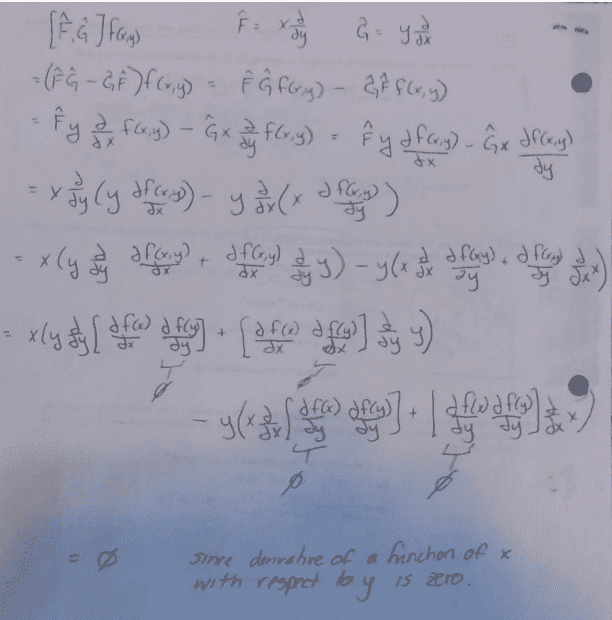# Help with this Commutator question please

Clifford Williams
Homework Statement:
Evaluate the commutator [𝑥(𝜕/𝜕𝑦), 𝑦(𝜕/𝜕𝑥)] by applying the operators to an arbitrary
function 𝑓(𝑥,𝑦).
Relevant Equations:
[A , B] = AB - BA
Hello,
In QM class this morning my Prof claimed that the commutator [𝑥(𝜕/𝜕𝑦), 𝑦(𝜕/𝜕𝑥)] = 0.
However, my classmate and I arrived at x(d/dx) - y(d/dy).
Can someone explain how (or if) our professor is correct?

Last edited:

ergospherical
\begin{align*}
[x \partial_y, y\partial_x] &= (x \partial_y)(y\partial_x) - (y\partial_x)(x\partial_y) \\
&= x(\partial_x + y \partial_{xy} ) - y(\partial_y + x \partial_{xy}) \\
&= x\partial_x - y\partial_y
\end{align*}

•wrobel and PeroK
Homework Helper
Gold Member
2022 Award
You can also check for a specific function, e.g, ##f(x, y) = x## to show that the commutator cannot be zero.

Last edited:
Clifford Williams
I checked a specific function, but I picked the function f(x) = x^2 +y^2, and my prof replied that "that's not a single valued function so it won't work, remember the postulates!"

But even when I plug in single valued functions s.a. y=x-x^3, the commutator is not 0...

My professor's solution uses the identity:
\begin{align*}

[\partial f(x,y)/\partial x] &= [\partial g(y)/\partial x]*[\partial h(x)/\partial x]

\end{align*}

and the fact that
\begin{align*}
[\partial h(y)/\partial x]=0
\end{align*}
to show that ∂f(x,y)/∂x goes to 0, and that the commutator is 0. Is this maybe for a special case?

One of those classes where I left more confused than I arrived.

Homework Helper
Gold Member
2022 Award
None of that makes any sense to me. At least one of you and the professor is confused.

•Clifford Williams
The problem was delivered exactly as phrased in the "Homework Statement":
"Evaluate the commutator [𝑥(𝜕/𝜕𝑦), 𝑦(𝜕/𝜕𝑥)] by applying the operators to an arbitrary
function 𝑓(𝑥,𝑦)."

That feeling that you're missing something? That's exactly where I've been all day...

ergospherical
Can you post your professor's solution? We can then look for the mistake.

Clifford Williams
My professor's solution is as follows:It relies on the identity
\begin{align*}

[\partial f(x,y)/\partial x] &= [\partial f(y)/\partial x]*[\partial f(x)/\partial x]

\end{align*}
to get from the penultimate line to the last line, however this is an identity I am neither familiar nor comfortable with.

Homework Helper
Gold Member
2022 Award
to get from the penultimate line to the last line, however this is an identity I am neither familiar nor comfortable with.
That's obviously wrong. In fact, what that "proves" is that ##x\frac{\partial}{\partial y} \equiv 0## and ##y\frac{\partial}{\partial x} \equiv 0##. That's because he's actually shown that each term in the commutator is separately zero. Which can't be right.

And, in fact, a simple corollary is that ##\frac{\partial}{\partial y} \equiv 0## and ##\frac{\partial}{\partial x} \equiv 0##. Which is a bizarre conclusion, to say the least.

Sometimes people, even professors, get things wrong.

•PhDeezNutz and ergospherical
PhDeezNutz
My professor's solution is as follows:
View attachment 297188
It relies on the identity
\begin{align*}

[\partial f(x,y)/\partial x] &= [\partial f(y)/\partial x]*[\partial f(x)/\partial x]

\end{align*}
to get from the penultimate line to the last line, however this is an identity I am neither familiar nor comfortable with.

To me that would imply every function ##f(x,y)## has a 0 derivative with respect to both ##x## and ##y##.

Staff Emeritus
Homework Helper
What did your professor mean by "that's not a single valued function so it won't work, remember the postulates!"?

•PeroK
Gold Member
2022 Award
It's proportional to
$$[\hat{x} \hat{p}_y,\hat{y} \hat{p}_x]=\hat{x}[\hat{p}_y,\hat{y} \hat{p}_x]+[\hat{x},\hat{y} \hat{p}_x]\hat{p}_y = \hat{x} [\hat{p}_y,\hat{y}] \hat{p}_x + \hat{y} [\hat{x},\hat{p}_x] \hat{p} y =-\mathrm{i} \hbar \hat{x} \hat{p}_x +\mathrm{i} \hbar \hat{y} \hat{p}_y \neq 0.$$
I've no clue, what's the meaning of the calculation presented as solution by the professor.

Homework Helper
Gold Member
2022 Award
My professor's solution is as follows:
View attachment 297188
It relies on the identity \begin{align*} [\partial f(x,y)/\partial x] &= [\partial f(y)/\partial x]*[\partial f(x)/\partial x]
\end{align*} to get from the penultimate line to the last line, however this is an identity I am neither familiar nor comfortable with.
f(x,y) magically changes from being a function of two variables to being a function of a single variable.

My professor's solution is as follows:
View attachment 297188
It relies on the identity
\begin{align*}

[\partial f(x,y)/\partial x] &= [\partial f(y)/\partial x]*[\partial f(x)/\partial x]

\end{align*}
to get from the penultimate line to the last line, however this is an identity I am neither familiar nor comfortable with.
Are you sure you copied it correctly?

LCSphysicist
This identity will give me nightmares.

Gold Member
2022 Award
Which identity? The obviously wrong formula quoted in #14? Just forget it. It's wrong. I've no clue, how one could come to the idea to write it down in the first place.

•PhDeezNutz
The original "identity" in post #4 is $$[\partial f(x,y)/\partial x] = [\partial g(y)/\partial x]*[\partial h(x)/\partial x]$$ By looking at it, one can assume that ##f(x,y)## is separable into ##h(x)## and ##g(y)##, i.e. ##f(x,y)=h(x)g(y)##.
*Edit: That comes from application of the product rule, $$[\partial f(x,y)/\partial x] = g(y)*[\partial h(x)/\partial x]+h(x)*[\partial g(y)/\partial x]=g(y)*[\partial h(x)/\partial x]+h(x)*0.$$
•